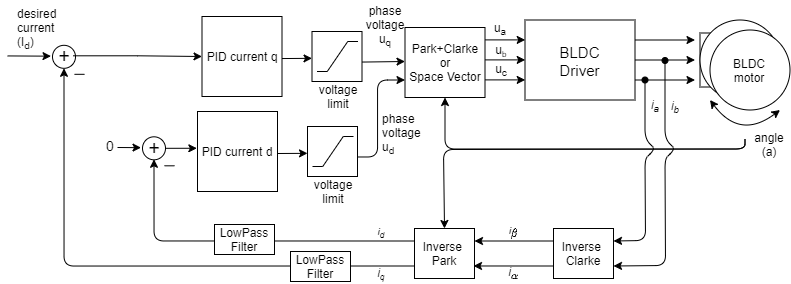# Torque control using FOC currents

This torque control mode allows you to the true torque control of a BLDC motor and it requires current sensing in order to do it. The user sets the target current Id to the FOC algorithm calculates the necessary phase voltages ua ,ub and uc in order to maintain it by measuring the phase currents (ia, ib and ic) and the rotor angle a. This mode is enabled by:

// FOC current torque control mode
motor.torque_controller = TorqueControlType::foc_current;


## How does it work exactlyThe FOC current torque control algorithm reads the phase currents of the BLDC motor (usually ia and ib). Furthermore the algorithm reads the rotor angle a from the position sensor. The phase currents are transformed into the d current id and q current iq using the Inverse Clarke and Park transform. Using the target current value Id and the measured currents iq and id, the PID controllers for each axis calculate the appropriate voltages Uq and Ud to be set to the motor, to maintain iq=Id and id=0. Finally using Park+Clarke (or SpaceVector) transformation the FOC algorithm sets the appropriate ua, ub and uc voltages to the motor. By measuring the phase currents this torque control algorithm ensures that these voltages generate the appropriate currents and magnetic force in the motor rotor with exactly 90 degree offset from its permanent magnetic field, which guarantees maximal torque, this is called commutation.

The torque generated in the motor is proportional to the q-axis current iq, making this torque control mode the true torque control of a BLDC motor.

## Configuration parameters

In order to make this loop run smoothly the user needs to configure the PID controller parameters of the PID_current_q and Low pass filter LPF_current_q time constant.

// Q axis
// PID parameters - default
motor.PID_current_q.P = 5;                       // 3    - Arduino UNO/MEGA
motor.PID_current_q.I = 1000;                    // 300  - Arduino UNO/MEGA
motor.PID_current_q.D = 0;
motor.PID_current_q.limit = motor.voltage_limit;
motor.PID_current_q.ramp = 1e6;                  // 1000 - Arduino UNO/MEGA
// Low pass filtering - default
LPF_current_q.Tf= 0.005;                         // 0.01 - Arduino UNO/MEGA

// D axis
// PID parameters - default
motor.PID_current_d.P = 5;                       // 3    - Arduino UNO/MEGA
motor.PID_current_d.I = 1000;                    // 300  - Arduino UNO/MEGA
motor.PID_current_d.D = 0;
motor.PID_current_d.limit = motor.voltage_limit;
motor.PID_current_d.ramp = 1e6;                  // 1000 - Arduino UNO/MEGA
// Low pass filtering - default
LPF_current_d.Tf= 0.005;                         // 0.01 - Arduino UNO/MEGA


## Torque control example code

A simple example of the FOC current based torque control using Inline current sensor and setting the target value by serial command interface.

#include <SimpleFOC.h>

// BLDC motor & driver instance
BLDCMotor motor = BLDCMotor(11);
BLDCDriver3PWM driver = BLDCDriver3PWM(9, 5, 6, 8);

// encoder instance
Encoder encoder = Encoder(2, 3, 500);
// channel A and B callbacks
void doA(){encoder.handleA();}
void doB(){encoder.handleB();}

// current sensor
InlineCurrentSense current_sense = InlineCurrentSense(0.01, 50.0, A0, A2);

// instantiate the commander
Commander command = Commander(Serial);
void doTarget(char* cmd) { command.scalar(&motor.target, cmd); }

void setup() {

// initialize encoder sensor hardware
encoder.init();
encoder.enableInterrupts(doA, doB);
// link the motor to the sensor

// driver config
// power supply voltage [V]
driver.voltage_power_supply = 12;
driver.init();

// current sense init hardware
current_sense.init();
// link the current sense to the motor

// set torque mode:
motor.torque_controller = TorqueControlType::foc_current;
// set motion control loop to be used
motor.controller = MotionControlType::torque;

// foc current control parameters (Arduino UNO/Mega)
motor.PID_current_q.P = 5;
motor.PID_current_q.I= 300;
motor.PID_current_d.P= 5;
motor.PID_current_d.I = 300;
motor.LPF_current_q.Tf = 0.01;
motor.LPF_current_d.Tf = 0.01;

// use monitoring with serial
Serial.begin(115200);
// comment out if not needed
motor.useMonitoring(Serial);

// initialize motor
motor.init();
// align sensor and start FOC
motor.initFOC();

Serial.println(F("Set the target current using serial terminal:"));
_delay(1000);
}

void loop() {

// main FOC algorithm function
motor.loopFOC();

// Motion control function
motor.move();

// user communication
command.run();
}